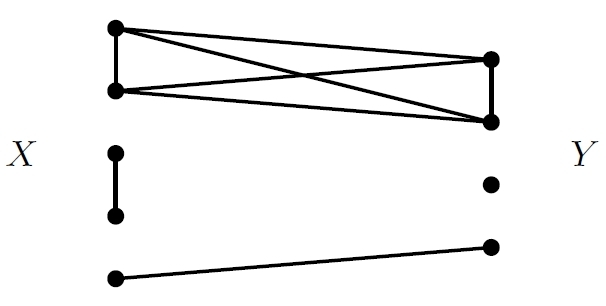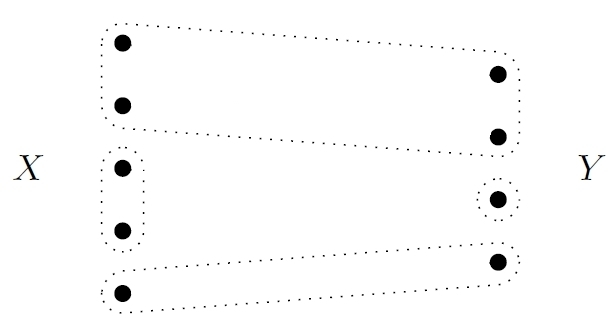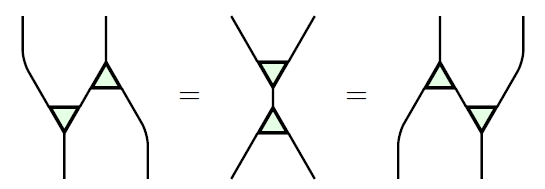## Corelations in Network Theory

Category theory reduces a large chunk of math to the clever manipulation of arrows. One of the fun things about this is that you can often take a familiar mathematical construction, think of it category-theoretically, and just turn around all the arrows to get something new and interesting!

In math we love functions. If we have a function$f: X \to Y$

we can formally turn around the arrow to think of$f$ as something going back from$Y$ back to$X$. But this something is usually not a function: it’s called a ‘cofunction’. A cofunction from$Y$ to$X$ is simply a function from$X$ to$Y.$

Cofunctions are somewhat interesting, but they’re really just functions viewed through a looking glass, so they don’t give much new—at least, not by themselves.

The game gets more interesting if we think of functions and cofunctions as special sorts of relations. A relation from$X$ to$Y$ is a subset$R \subseteq X \times Y$

It’s a function when for each$x \in X$ there’s a unique$y \in Y$ with$(x,y) \in R.$ It’s a cofunction when for each$y \in Y$ there’s a unique$x \in x$ with$(x,y) \in R.$

Just as we can compose functions, we can compose relations. Relations have certain advantages over functions: for example, we can ‘turn around’ any relation$R$ from$X$ to$Y$ and get a relation$R^\dagger$ from$Y$ to$X:$$R^\dagger = \{(y,x) : \; (x,y) \in R \}$

If we turn around a function we get a cofunction, and vice versa. But we can also do other fun things: for example, since both functions and cofunctions are relations, we can compose a function and a cofunction and get a relation.

Of course, relations also have certain disadvantages compared to functions. But it’s utterly clear by now that the category$\mathrm{FinRel},$ where the objects are finite sets and the morphisms are relations, is very important.

So far, so good. But what happens if we take the definition of ‘relation’ and turn all the arrows around?

There are actually several things I could mean by this question, some more interesting than others. But one of them gives a very interesting new concept: the concept of ‘corelation’. And two of my students have just written a very nice paper on corelations:

• Brandon Coya and Brendan Fong, Corelations are the prop for extraspecial commutative Frobenius monoids.

Here’s why this paper is important for network theory: corelations between finite sets are exactly what we need to describe electrical circuits made of ideal conductive wires! A corelation from a finite set$X$ to a finite set$Y$ can be drawn this way:I have drawn more wires than strictly necessary: I’ve drawn a wire between two points whenever I want current to be able to flow between them. But there’s a reason I did this: a corelation from$X$ to$Y$ simply tells us when current can flow from one point in either of these sets to any other point in these sets.

Of course circuits made solely of conductive wires are not very exciting for electrical engineers. But in an earlier paper, Brendan introduced corelations as an important stepping-stone toward more general circuits:

• John Baez and Brendan Fong, A compositional framework for passive linear circuits. (Blog article here.)

The key point is simply that you use conductive wires to connect resistors, inductors, capacitors, batteries and the like and build interesting circuits—so if you don’t fully understand the math of conductive wires, you’re limited in your ability to understand circuits in general!

In their new paper, Brendan teamed up with Brandon Coya, and they figured out all the rules obeyed by the category$\mathrm{FinCorel},$ where the objects are finite sets and the morphisms are corelations. I’ll explain these rules later.

This sort of analysis had previously been done for$\mathrm{FinRel},$ and it turns out there’s a beautiful analogy between the two cases! Here is a chart displaying the analogy:

 Spans Cospans extra bicommutative bimonoids special commutative Frobenius monoids Relations Corelations extraspecial bicommutative bimonoids extraspecial commutative Frobenius monoids

I’m sure this will be cryptic to the nonmathematicians reading this, and even many mathematicians—but the paper explains what’s going on here.

I’ll actually say what an ‘extraspecial commutative Frobenius monoid’ is later in this post. This is a terse way of listing all the rules obeyed by corelations between finite sets—and thus, all the rules obeyed by conductive wires.

But first, let’s talk about something simpler.

### What is a corelation?

Just as we can define functions as relations of a special sort, we can also define relations in terms of functions. A relation from$X$ to$Y$ is a subset$R \subseteq X \times Y$

but we can think of this as an equivalence class of one-to-one functions$i: R \to X \times Y$

Why an equivalence class? The image of$i$ is our desired subset of$X \times Y.$ The set$R$ here could be replaced by any isomorphic set; its only role is to provide ‘names’ for the elements of$X \times Y$ that are in the image of$i.$

Now we have a relation described as an arrow, or really an equivalence class of arrows. Next, let’s turn the arrow around!

There are different things I might mean by that, but we want to do it cleverly. When we turn arrows around, the concept of product (for example, cartesian product$X \times Y$ of sets) turns into the concept of sum (for example, disjoint union$X + Y$ of sets). Similarly, the concept of monomorphism (such as a one-to-one function) turns into the concept of epimorphism (such as an onto function). If you don’t believe me, click on the links!

So, we should define a corelation from a set$X$ to a set$Y$ to be an equivalence class of onto functions$p: X + Y \to C$

Why an equivalence class? The set$C$ here could be replaced by any isomorphic set; its only role is to provide ‘names’ for the sets of elements of$X + Y$ that get mapped to the same thing via$p.$

In simpler terms, a corelation from$X$ to a set$Y$ is just a partition of the disjoint union$X + Y.$ So, it looks like this:If we like, we can then draw a line connecting any two points that lie in the same part of the partition:These lines determine the corelation, so we can also draw a corelation this way:This is why corelations describe circuits made solely of wires!

### The rules governing corelations

The main result in Brandon and Brendan’s paper is that$\mathrm{FinCorel}$ is equivalent to the PROP for extraspecial commutative Frobenius monoids. That’s a terse way of the laws governing$\mathrm{FinCorel}.$

Let me just show you the most important laws. In each of these law I’ll draw two circuits made of wires, and write an equals sign asserting that they give the same corelation from a set$X$ to a set$Y.$ The inputs$X$ of each circuit are on top, and the outputs$Y$ are at the bottom. I’ll draw 3-way junctions as little triangles, but don’t worry about that. When we compose two corelations we may get a wire left in mid-air, not connected to the inputs or outputs. We draw the end of the wire as a little circle.

There are some laws called the ‘commutative monoid’ laws:and an upside-down version called the ‘cocommutative comonoid’ laws:Then we have ‘Frobenius laws’:and finally we have the ‘special’ and ‘extra’ laws:All other laws can be derived from these in some systematic ways.

Commutative Frobenius monoids obey the commutative monoid laws, the cocommutative comonoid laws and the Frobenius laws. They play a fundamental role in 2d topological quantum field theory. Special Frobenius monoids are also well-known. But the ‘extra’ law, which says that a little piece of wire not connected to anything can be thrown away with no effect, is less well studied. Jason Erbele and I gave it this name in our work on control theory:

• John Baez and Jason Erbele, Categories in control. (Blog article here.)

### For more

David Ellerman has spent a lot of time studying what would happen to mathematics if we turned around a lot of arrows in a certain systematic way. In particular, just as the concept of relation would be replaced by the concept of corelation, the concept of subset would be replaced by the concept of partition. You can see how it fits together: just as a relation from$X$ to$Y$ is a subset of$X \times Y,$ a corelation from$X$ to$Y$ is a partition of$X + Y.$

There’s a lattice of subsets of a set:In logic these subsets correspond to propositions, and the lattice operations are the logical operations ‘and’ and ‘or’. But there’s also a lattice of partitions of a set:In Ellerman’s vision, this lattice of partitions gives a new kind of logic. You can read about it here:

• David Ellerman, Introduction to partition logic, Logic Journal of the Interest Group in Pure and Applied Logic 22 (2014), 94–125.

As mentioned, the main result in Brandon and Brendan’s paper is that$\mathrm{FinCorel}$ is equivalent to the PROP for extraspecial commutative Frobenius monoids. After they proved this, they noticed that the result has also been stated in other language and proved in other ways by two other authors:

• Fabio Zanasi, Interacting Hopf Algebras—the Theory of Linear Systems, PhD thesis, École Normale Supériere de Lyon, 2015.

• K. Dosen and Z. Petrić, Syntax for split preorders, Annals of Pure and Applied Logic 164 (2013), 443–481.

Unsurprisingly, I prefer Brendan and Brandon’s approach to deriving the result. But it’s nice to see different perspectives!

### 16 Responses to Corelations in Network Theory

1.Qiaochu Yuan says:

Cool. But really not a fan of this term “corelation” because it’s way too easy to read as a misspelling of “correlation.” A new term unrelated to the term “relation” would probably have been fine. (I think mathematicians as a rule overrate the importance of systematic terminology, relative to e.g. physicists; that’s the kind of thinking that would’ve given the world “Lorentzian singularity” or something instead of “black hole.”)

•sma045 says:

Such problems in English can be resolved with hyphenation (“co-relation”), though some people hate that. This would work better if software wouldn’t put line breaks after hyphens.

•John Baez says:

Unfortunately the term “corelation” is reasonably well established, and none of the people using it have any desire to stop!

•Todd Trimble says:

I also support co-relation (with the hyphen).

•David Ellerman says:

Hyphen, no-hyphen; mox nix to me

•jvm says:

Who thought like me that “corelation” was a typo and then felt a bit stupid…? :D

I became a big fan of the term because it taught me something: 1) read carefully before thinking someone’s wrong and 2) beware of “co”s in category theory!

2.Eugene says:

I enjoyed reading the post. I also appreciate the links to various references. I presume Brendan and you have plans for this technology. Or am I being presumptuous?

•John Baez says:

You’re not being presumptuous—at least, not incorrectly presumptuous. Brendan and I already needed corelations in our paper on a compositional framework for passive linear networks, but this ‘generators and relations’ description of a PROP equivalent to the symmetric monoidal category of corelations will play a part in a new paper we’re writing with Brandon Coya and Franciscus Rebro. Jason Erbele’s thesis on control theory will also use PROPs. The overall strategy is to describe various kinds of networks showing up in applied mathematics as morphisms in decorated cospan categories, but also give general and relations descriptions of PROPs equivalent to these decorated cospan categories.

3.Graham says:

This post has the same effect on me as the one on operads and phylogenetic trees. It looks familiar but I don’t understand it because I don’t understand category theory.

The most important partitions that occur in my work are partitions of individual organisms into sets called ‘species’. I often think of these partitions in terms of trees. In the diagram of the lattice of partitions of a set, each path from any node X up to the top represents a binary tree whose tips are the subsets in the partition at X.

4.Fabio Gadducci says:

Dear John, nice blog entry. You might be interested to the fact that we investigated which algebraic laws hold for spans and cospans with respect to cartesian product and disjoint union, tracing the connection with various kinds of relations, in two old articles, namely

 R. Bruni, F. Gadducci: Some algebraic laws for spans. RelMiS 2001, ENTCS 44(3), 175-193
 R. Bruni, F. Gadducci, U. Montanari: Normal forms for algebras of connections. TCS 286(2): 247-292 (2002)

 discusses the interplay between the two monoidal operators and the span/cospan dichotomy over Set, while  basically discusses the same situation, yet wrt. Graph.

More to the point, it seems to me that in  we call equivalence relations what you call corelations, and we have the same extra and special laws. Check e.g. Table 1, axioms 1 and 5.

I will try and better understand your results, in order to trace a precise comparison. Best, Fabio

•John Baez says:

Thanks! I’ll take a look at your papers. Actually Brendan and Brandon’s paper cites the first of the two papers you mentioned here. I talked to Brendan and he said you list all the laws implicit in the phrase ‘extraspecial commutative Frobenius monoid’, and prove that$\mathrm{FinCorel}$ obeys these laws, but don’t prove that all the laws obeyed by$\mathrm{FinCorel}$ can be derived from these. If so, you essentially got a symmetric monoidal functor from the PROP for extraspecial commutative Frobenius monoids to$\mathrm{FinCorel},$ but didn’t prove it’s an equivalence.

•Fabio Gadducci says:

Indeed, in  we just proved the soudness of those laws, so to say, as part of a taxonomy of span/cospan categories over Set. A more precise correspondence statement is in , even if the notation there is admittedly a bit unwieldy: see Propositions 4.8 and 4.9, the latter being the completeness bit, which is actually just exploiting the older paper

 V.-E. Cazanescu, Gh. Stefanescu: Classes of finite relations as initial abstract data types I. Discrete Mathematics 90: 233–265 (1991)

I am very excited by the novel applications and connections that you are pushing, and looking forward to more results in the future.

5. At some point I gave Brendan Fong a project: describe the category whose morphisms are electrical circuits. He took up the challenge much more ambitiously than I’d ever expected, developing powerful general frameworks to solve not only this problem but also many others. He did this in a number of papers, most of which I’ve already discussed […]

6. Yesterday, Marco Grandis showed me a fascinating paper by his thesis advisor:

• G. Darbo, Aspetti algebrico-categoriali della teoria dei dispotivi, Symposia Mathematica IV (1970), Istituto Nazionale di Alta Matematica, 303–336.

It’s closely connected to Brendan Fong’s thesis, but also different—and, of course, much older. According to Grandis, Darbo was the first person to work on category theory in Italy. He’s better known for other things, like ‘Darbo’s fixed point theorem’, but this piece of work is elegant, and, it seems to me, strangely ahead of its time.

The paper’s title translates as ‘Algebraic-categorical aspects of the theory of devices’, and its main concept is that of a ‘universe of devices’: a collection of devices of some kind that can be hooked up using wires to form more devices of this kind. Nowadays we might study this concept using operads—but operads didn’t exist in 1970, and Darbo did quite fine without them.

The key is the category$\mathrm{FinCorel},$ which has finite sets as objects and ‘corelations’ as morphisms. I explained corelations here:

Corelations in network theory, 2 February 2016.

7. In this paper, we study the props for various kinds of electrical circuits:

• John Baez, Brandon Coya and Franciscus Rebro, Props in network theory.

[…]

In Section 5 we discuss presentations of props. Proposition 19, proved in Appendix A.2 using a result of Todd Trimble, shows that the category of props is monadic over the category of signatures,$\mathrm{Set}^{\mathbb{N} \times \mathbb{N}}.$ This lets us work with props using generators and relations. We conclude by recalling a presentation of$\mathrm{FinCospan}$ due to Lack and a presentation of$\mathrm{FinCorel}$ due to Coya and Fong:

• Steve Lack, Composing PROPs, Theory and Applications of Categories 13 (2004), 147–163.

• Brandon Coya and Brendan Fong, Corelations are the prop for extraspecial commutative Frobenius monoids, Theory and Applications of Categories 32 (2017), 380–395. (Blog article here.)

8. My student Brandon Coya has finished his thesis!

• Brandon Coya, Circuits, Bond Graphs, and Signal-Flow Diagrams: A Categorical Perspective, Ph.D. thesis, U. C. Riverside, 2018.

It’s about networks in engineering. He uses category theory to study the diagrams engineers like to draw, and functors to understand how these diagrams are interpreted.

His thesis raises some really interesting pure mathematical questions about the category of corelations and a ‘weak bimonoid’ that can be found in this category. Weak bimonoids were invented by Pastro and Street in their study of ‘quantum categories’, a generalization of quantum groups. So, it’s fascinating to see a weak bimonoid that plays an important role in electrical engineering!

However, in what follows I’ll stick to less fancy stuff: I’ll just explain the basic idea of Brandon’s thesis, say a bit about circuits and ‘bond graphs’, and outline his main results.

This site uses Akismet to reduce spam. Learn how your comment data is processed.